#### Tagpython

I’ve been getting more into theoretical/analytical modeling lately, and I’ve been playing around with software to help me do some of the more complicated calculus involved (for two reasons: 1) I’m lazy, 2) My skills are very rusty). Python (of course) provides excellent symbolic capabilities through the Sympy module. I played around with it to try and get a feel for how it works, but I couldn’t find any help online, nor anyone who has posted a tutorial, on analyzing basic biological/ecological models with Sympy. So here is my version. Below, I solve both the exponential growth and logistic growth models using Sympy, then plot the results. Here is a step-by-step tutorial for Bio-Sympy.

Exponential Growth

Exponential growth is defined by the differential equation

$$\frac{dy(t)}{dt} = k*y(t)$$

and this ODE has the analytical solution of

$$y(t) = Y_0 e^{kt}$$

So how do we use Sympy to go from the ODE to the general solution? Well, like this.

First, gotta import the modules we need, and then initialize pretty printing just so the output is readable (see the Sympy docs for this):

import sympy as sm
import numpy as np
import matplotlib.pyplot as plt
sm.init_printing()

Next, define the variables k (the intrinsic growth rate) and t (for time). Then, make y a function of t

from sympy.abc import t, k
y = sm.Function('y')(t)

Then, define the derivative of y with respect to t (the left-hand side of the ODE), and then define the right-hand side of the ODE.

dy = y.diff(t)
rhs = k*y

We need to set these two quantities equal to one another, as in the ODE above, which we can do using a Sympy Equality

eq = sm.Eq(dy, rhs)

If you print eq, it should give you the differential equation. Now that we have the differential equation set up, all we need to do is solve. Since this is a simple one, Sympy can do it on its own with no hints or guesses:

sol = sm.dsolve(eq)
sol

Where sol should give you the analytical solution $$y(t) = C_1 e^{kt}$$. But $$C_1$$ here is just a constant, we want to put it in terms of the initial conditions. (I know this is trivial in this case, but bear with me). First, we need to find the initial conditions. We do so by substituting 0 in for t, and then setting that equal to n0.

t0 = sol.args.subs({'t': 0})
n0 = sm.symbols('n0')
eq_init = sm.Eq(n0, t0)

The object eq_init should now be $$n_0 = C_1$$. Great. Now, solve that in terms of $$C_1$$ and substitute it back into the original equation.

C1 = eq_init.args # this chunk just isolates the C1 variable as a symbol so we can use it in Sympy
sm.solve(eq_init, C1)
init_solve= sm.solve(eq_init, C1)
final = sol.subs(C1, init_solve)

final is now $$y(t) = n_0 e^{kt}$$. Just like it should be! I’ll leave plotting that one up to you.

Logistic Growth

Logistic growth is defined by the differential equation

$$\frac{dy(t)}{dt} = ry(t)\left ( 1 -\frac{y(t)}{K} \right )$$

The steps for solving this are identical to the steps above:

# define the needed parameters
from sympy.abc import r, K, t
y = sm.Function('y')(t)
# define the differential equation
dy = y.diff(t)
rhs = r*y*(1 - y/K)
# define the equality
eq = sm.Eq(dy, rhs)
# find the general solution
sol = sm.dsolve(eq))

sol is now more complex:

$$y(t) = \frac{Ke^{C_1 K + rt}}{e^{C_1 K + rt} – 1}$$
# find out what C is in terms of y0
t0 = sol.args.subs({'t':0})
n0 = sm.symbols('n0')
eq_init = sm.Eq(n0, t0)
# it takes a little more work to isolate C1 here
# try each step for yourself to see what it does
C1 = t0.args.args.args
t0_sol = sm.solve(eq_init, C1)

The initial conditions are also rather complex:

$$C_1 =\frac{\log \left ( \frac{-n_0)}{K-n_0} \right )}{K}$$

But we can substitute that back into the original solution to get the general solution in terms of the initial conditions

# substitute the expression for C1 back into the equation
final = sol.args.subs(C1, t0_sol)

where final is now

$$y(t) = \frac{-K n_0 e^{rt}}{ (K-n_0) \left ( – \frac{n_0 e^{rt}}{K-n_0} – 1 \right ) }$$

Holy cow! That’s a doozy, and it doesn’t look anything like the general solutions you’ll find elsewhere. Easy fix, just ask Sympy to simplify it!

final.simplify()

and we have

$$y(t) = \frac{ K n_0 e^{rt} }{ K + n_0 e^{rt} – n_0 }$$

which we can easily simplify further in the denominator ourselves:

$$y(t) = \frac{ K n_0 e^{rt} }{ K + n_0 (e^{rt} -1) }$$

and we have the general solution to logistic growth that everyone knows and love!

Viola, Sympy for solving biological ODEs

As described in an earlier post, Gaussian process models are a fast, flexible tool for making predictions. They’re relatively easy to program if you happen to know the parameters of your covariance function/kernel, but what if you want to estimate them from the data? There are several methods available, but my favorite so far is STAN. True, it requires programming the kernel by hand, but I actually find this easier to understand than trying to parse out the kernel functions from, say, scikit-learn.

STAN can fit GP models quickly, but there are certain tricks you can do that make it lightning fast and accurate. I’ve had trouble getting scikit to converge on a stable/accurate solution, but STAN does this with no problem. Plus, the Hamiltonian Monte-Carlo sampler is very quick for GPs (see the STAN User Manual for more).

Here’s a quick tutorial on how to fit GPs in STAN, and how to speed them up. First, let’s import our modules and simulate some fake data:

import numpy as np
import scipy.stats as st
import matplotlib.pyplot as plt

X = np.arange(-5, 6)
Y_m = np.sin(X)
Y = Y_m + np.random.normal(0, 0.5, len(Y_m))

Now, let’s develop the STAN model. Here’s the boilerplate model for a GP using a squared-exponential model (taken right from the STAN manual).

gp_no_pred = """
data{
int<lower=1> N;
vector[N] X;
vector[N] Y;
}
transformed data{
vector[N] mu;

for(n in 1:N) mu[n] = 0;
}
parameters{
real<lower=0> s_f;
real<lower=0> inv_rho;
real<lower=0> s_n;
}
transformed parameters{
real<lower=0> rho;

rho = inv(inv_rho);
}
model{
matrix[N,N] Sigma;

for(i in 1:(N-1)){
for(j in (i+1):N){
Sigma[i,j] = s_f * exp(-rho * pow(X[i]-X[j], 2));
Sigma[j,i] = Sigma[i,j];
}
}

for(k in 1:N){
Sigma[k,k] = s_f + s_n;
}

Y ~ multi_normal(mu, Sigma);
s_f ~ cauchy(0,5);
inv_rho ~ cauchy(0,5);
s_n ~ cauchy(0,5);

}
"""

This model fits the parameters of the kernel. On my computer, it does so in about  0.15 seconds total (1000 iterations).

Now that we know what the hyperparameters are, we’d like to predict new values, but we also want to do so incorporating full uncertainty in the hyperparameters. The way the STAN manual says to go about this is to make a second vector containing the locations you’d like to predict, paste those together to the X values you have, make a vector of prediction points as parameters, paste those to the Y values you have, and feed those into the multivariate normal distribution as one big mush. The issue here is that the covariance matrix, Sigma, gets very large very fast. Large covariance matrices take a while to invert in the multivariate probability density, and so slows down the sampler.

Here’s the model, after making a vector of 100 prediction points to get a smooth line:

X_pred = np.linspace(-5, 7, 100)

gp_pred1 = """
data{
int<lower=1> N1;
int<lower=1> N2;
vector[N1] X;
vector[N1] Y;
vector[N2] Xp;
}
transformed data{
int<lower=1> N;
vector[N1+N2] Xf;
vector[N1+N2] mu;

N = N1+N2;
for(n in 1:N) mu[n] = 0;
for(n in 1:N1) Xf[n] = X[n];
for(n in 1:N2) Xf[N1+n] = Xp[n];
}
parameters{
real<lower=0> s_f;
real<lower=0> inv_rho;
real<lower=0> s_n;
vector[N2] Yp;
}
transformed parameters{
real<lower=0> rho;

rho = inv(inv_rho);
}
model{
matrix[N,N] Sigma;
vector[N] Yf;

for(n in 1:N1) Yf[n] = Y[n];
for(n in 1:N2) Yf[N1+n] = Yp[n];

for(i in 1:(N-1)){
for(j in (i+1):N){
Sigma[i,j] = s_f * exp(-rho * pow(Xf[i]-Xf[j], 2));
Sigma[j,i] = Sigma[i,j];
}
}

for(k in 1:N){
Sigma[k,k] = s_f + s_n;
}

Yf ~ multi_normal(mu, Sigma);

s_f ~ cauchy(0,5);
inv_rho ~ cauchy(0,5);
s_n ~ cauchy(0, 5);
}
"""

This is extremely slow, taking about 81 seconds on my computer (up from less than one second). Taking the cholesky decompose of Sigma an using multi_normal_cholesky didn’t speed things up, either.

We can speed this up by taking advantage of the analytical form of the solution. That is, once we know the hyperparameters of the kernel from the observed data, we can directly calculate the multivariate normal distribution of the predicted data:

$$P(Y_p | X_p, X_o, Y_o) \sim Normal(\boldsymbol{K}_{obs}^{*’} \boldsymbol{K}_{obs}^{-1} \boldsymbol{y}_{obs}, \boldsymbol{K}^{*} – \boldsymbol{K}_{obs}^{*’} \boldsymbol{K}_{obs}^{-1} \boldsymbol{K}_{obs}^{*})$$

We can calculate those quantities directly within STAN. Then, as an added trick, we can take advantage of the Cholesky decomposition to generate random samples of $$Y_p$$ within STAN as well. Here’s the annotated model:

data{
int<lower=1> N1;
int<lower=1> N2;
vector[N1] X;
vector[N1] Y;
vector[N2] Xp;
}
transformed data{
vector[N1] mu;

for(n in 1:N1) mu[n] = 0;
}
parameters{
real<lower=0> s_f;
real<lower=0> inv_rho;
real<lower=0> s_n;
// This is going to be just a generic (0,1) vector
// for use in generating random samples
vector[N2] z;
}
transformed parameters{
real<lower=0> rho;

rho = inv(inv_rho);
}
model{
// kernel for only the observed data
matrix[N1,N1] Sigma;

for(i in 1:(N1-1)){
for(j in (i+1):N1){
Sigma[i,j] = s_f * exp(-rho * pow(X[i]-X[j], 2));
Sigma[j,i] = Sigma[i,j];
}
}

for(k in 1:N1){
Sigma[k,k] = s_f + s_n;
}

// sampling statement for only the observed data
Y ~ multi_normal(mu, Sigma);
// generic sampling statement for z
z ~ normal(0,1);

s_f ~ cauchy(0,5);
inv_rho ~ cauchy(0,5);
s_n ~ cauchy(0, 5);
}
generated quantities{
matrix[N1,N1] Ko;
matrix[N2,N2] Kp;
matrix[N1,N2] Kop;
matrix[N1,N1] L;
matrix[N1, N1] Ko_inv;
vector[N2] mu_p;
matrix[N2,N2] Tau;
vector[N2] Yp;
matrix[N2,N2] L2;

// kernel for observed data
for(i in 1:(N1-1)){
for(j in (i+1):N1){
Ko[i,j] = s_f * exp(-rho * pow(X[i]-X[j], 2));
Ko[j,i] = Ko[i,j];
}
}

for(k in 1:N1){
Ko[k,k] = s_f + s_n;
}

// kernel for prediction data
for(i in 1:(N2-1)){
for(j in (i+1):N2){
Kp[i,j] = s_f * exp(-rho * pow(Xp[i]-Xp[j], 2));
Kp[j,i] = Kp[i,j];
}
}

for(k in 1:N2){
Kp[k,k] = s_f + s_n;
}

// kernel for observed-prediction cross
for(i in 1:N1){
for(j in 1:N2){
Kop[i,j] = s_f * exp(-rho * pow(X[i]-Xp[j], 2));
}
}

// Follow the algorithm 2.1 of Rassmussen and Williams
// cholesky decompose Ko
L = cholesky_decompose(Ko);
Ko_inv = inverse(L') * inverse(L);
// calculate the mean of the Y prediction
mu_p = Kop' * Ko_inv * Y;
// calculate the covariance of the Y prediction
Tau = Kp - Kop' * Ko_inv * Kop;

// Generate random samples from N(mu,Tau)
L2 = cholesky_decompose(Tau);
Yp = mu_p + L2*z;

}
"""

This model runs at 3.5 seconds, huge improvement over 81. If you want the predictions, just extract Yp, and that gives you predictions with full uncertainty. We can also compare the two outputs:

Note: I have no idea why, but upping the iterations from 1000 to 5000 causes the analytical solution model to freeze my computer. I can’t quite figure that one out.

Gaussian Processes for Machine Learning by Rasmussen and Williams has become the quintessential book for learning Gaussian Processes. They kindly provide their own software that runs in MATLAB or Octave in order to run GPs. However, I find it easiest to learn by programming on my own, and my language of choice is Python. This is the first in a series of posts that will go over GPs in Python and how to produce the figures, graphs, and results presented in Rasmussen and Williams.

• Note, Python as numerous excellent packages for implementing GPs, but here I will work on doing them myself “by hand”.

This post will cover the basics presented in Chapter 2. Specifically, we will cover Figures 2.2, 2.4, and 2.5.

• Note, I’m not covering the theory of GPs here (that’s the subject of the entire book, right?)

Before we get going, we have to set up Python:

import numpy as np
import scipy.stats as st
import matplotlib.pyplot as plt

# Figure 2.2

We want to make smooth lines to start, so make 100 evenly spaced $$x$$ values:

N_star = 101
x_star = np.linspace(-5, 5, N_star)

Next we have to calculate the covariances between all the observations and store them in the matrix $$\boldsymbol{K}$$. Here, we use the squared exponential covariance: $$\text{exp}[-\frac{1}{2}(x_i – x_j)^2]$$

K_star = np.empty((N_star, N_star))
for i in range(N_star):
for j in range(N_star):
K_star[i,j] = np.exp(-0.5 * (x_star[i] - x_star[j])**2)

We now have our prior distribution with a mean of 0 and a covariance matrix of $$\boldsymbol{K}$$. We can draw samples from this prior distribution

priors = st.multivariate_normal.rvs(mean=*N_star, cov=K_star, size=1000)

for i in range(5):
plt.plot(x_star, priors[i])

plt.fill_between(x_star, np.percentile(priors, 2.5, axis=0), np.percentile(priors, 97.5, axis=0), alpha=0.2)
plt.show()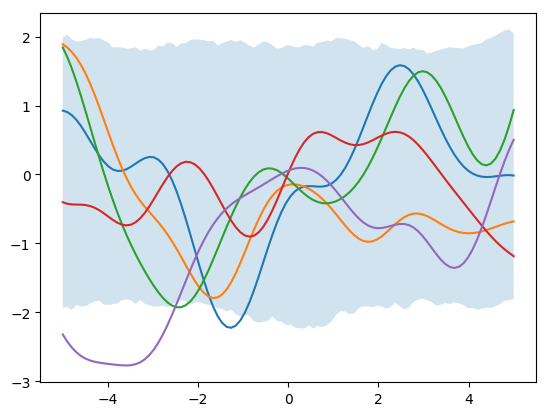Next, let’s add in some observed data:

x_obs = np.array([-4.5, -3.5, -0.5, 0, 1])
y_obs = np.array([-2, 0, 1, 2, -1])

We now need to calculate the covariance between our unobserved data (x_star) and our observed data (x_obs), as well as the covariance among x_obs points as well. The first for loop calculates observed covariances. The second for loop calculates observed-new covariances.

N_obs = 5
K_obs = np.empty((N_obs, N_obs))
for i in range(N_obs):
for j in range(N_obs):
K_obs[i,j] = np.exp(-0.5 * (x_obs[i] - x_obs[j])**2)

K_obs_star = np.empty((N_obs, N_star))
for i in range(N_obs):
for j in range(N_star):
K_obs_star[i,j] = np.exp(-0.5*(x_obs[i] - x_star[j])**2)

We can then get our posterior distributions:

$$\boldsymbol{\mu} = \boldsymbol{K}_{obs}^{*’} \boldsymbol{K}_{obs}^{-1} \boldsymbol{y}_{obs}$$
$$\boldsymbol{\Sigma} = \boldsymbol{K}^{*} – \boldsymbol{K}_{obs}^{*’} \boldsymbol{K}_{obs}^{-1} \boldsymbol{K}_{obs}^{*}$$

and simulate from this posterior distribution.

post_mean = K_obs_star.T.dot(np.linalg.pinv(K_obs)).dot(y_obs)
post_var = K_star - K_obs_star.T.dot(np.linalg.pinv(K_obs)).dot(K_obs_star)

posteriors = st.multivariate_normal.rvs(mean=post_mean, cov=post_var, size=1000)

for i in range(1000):
plt.plot(x_star, posteriors[i], c='k', alpha=0.01)

plt.plot(x_obs, y_obs, 'ro')
plt.show()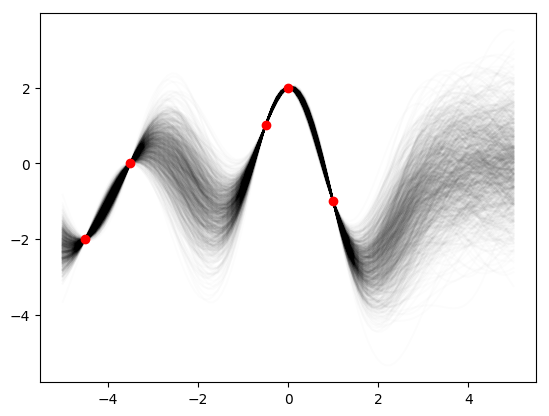This may not look exactly like the Rasmussen and Williams Fig. 2.2b because I guessed at the data points and they may not be quite right. As the authors point out, we can actually plot what the covariance looks like for difference x-values, say $$x=-1,2,3$$.

plt.plot(x_star, post_var, label='x= = -2')
plt.plot(x_star, post_var, label='x = 1')
plt.plot(x_star, post_var, label='x = 3')
plt.ylabel('Posterior Covariance')
plt.legend()
plt.show()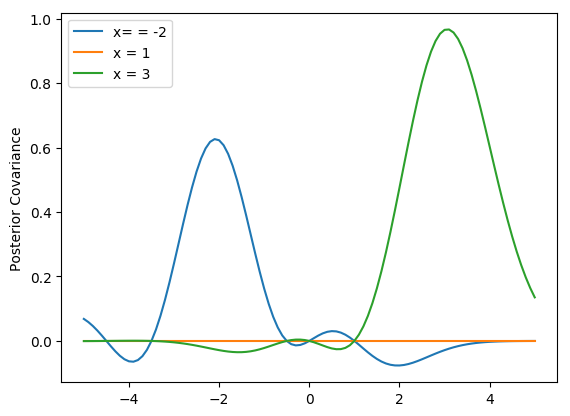In this case, however, we’ve forced the scale to be equal to 1, that is you have to be at least one unit away on the x-axis before you begin to see large changes $$y$$. We can incorporate a scale parameter $$\lambda$$ to change that. We can use another parameter $$\sigma_f^2$$ to control the noise in the signal (that is, how close to the points does the line have to pass) and we can add further noise by assuming measurement error $$\sigma_n^2$$.

$$cov(x_i, x_j) = \sigma_f^2 \text{exp}[-\frac{1}{2\lambda^2} (x_i – x_j)^2] + \delta_{ij}\sigma_n^2$$

Let’s make some new data:

x_obs = np.array([-7, -5.5, -5, -4.8, -3, -2.8, -1.5,
-1, -0.5, 0.25, 0.5, 1, 2.5, 2.6, 4.5, 4.6, 5, 5.5, 6])
y_obs = np.array([-2, 0, 0.1, -1, -1.2, -1.15, 0.5, 1.5, 1.75,
0, -0.9, -1, -2.5, -2, -1.5, -1, -1.5, -1.1, -1])
x_star = np.linspace(-7, 7, N_star)

Next, make a couple of functions to calculate $$\boldsymbol{K}_{obs}$$, $$\boldsymbol{K}^{*}$$, and $$\boldsymbol{K}_{obs}^{*}$$.

def k_star(l, sf, sn, x_s):
N_star = len(x_s)
K_star = np.empty((N_star, N_star))
for i in range(N_star):
for j in range(N_star):
K_star[i,j] = sf**2*np.exp(-(1.0 / (2.0*l**2)) * (x_s[i] - x_s[j])**2)
return K_star

def k_obs(l, sf, sn, x_o):
N_obs = len(x_o)
K_obs = np.empty((N_obs, N_obs))
for i in range(N_obs):
for j in range(N_obs):
K_obs[i,j] = sf**2*np.exp(-(1.0 / (2.0*l**2)) * (x_o[i] - x_o[j])**2)
for i in range(N_obs):
K_obs[i,i] += sn**2
return K_obs

def k_obs_star(l, sf, sn, x_o, x_s):
N_obs = len(x_o)
N_star = len(x_s)
K_obs_star = np.empty((N_obs, N_star))
for i in range(N_obs):
for j in range(N_star):
K_obs_star[i,j] = sf**2*np.exp(-(1.0 / (2.0*l**2)) *(x_o[i] - x_s[j])**2)
return K_obs_star

Then run the code for the various sets of parameters. Let’s start with (1, 1, 0.1):

K_star = k_star(1,1,0.2,x_star)
K_obs = k_obs(1,1,0.2, x_obs)
K_obs_star = k_obs_star(1,1,0.2,x_obs, x_star)

post_mean = K_obs_star.T.dot(np.linalg.pinv(K_obs)).dot(y_obs)
post_var = K_star - K_obs_star.T.dot(np.linalg.pinv(K_obs)).dot(K_obs_star)

posteriors = st.multivariate_normal.rvs(mean=post_mean, cov=post_var, size=1000)

for i in range(1000):
plt.plot(x_star, posteriors[i], c='k', alpha=0.01)

plt.plot(x_star, posteriors.mean(axis=0))
plt.plot(x_obs, y_obs, 'ro')
plt.show()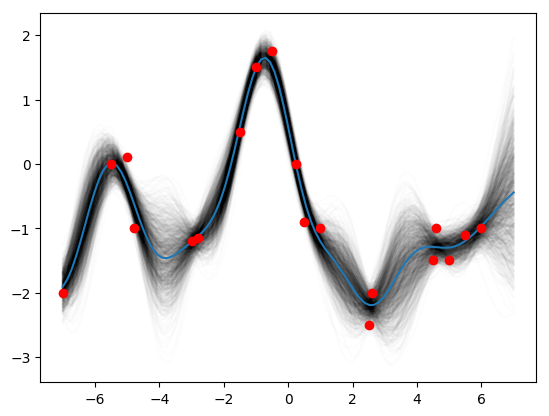Then try (0.3, 1.08, 0.00005):

K_star = k_star(0.3, 1.08, 0.00005, x_star)
K_obs = k_obs(0.3, 1.08, 0.00005,  x_obs)
K_obs_star = k_obs_star(0.3, 1.08, 0.00005, x_obs, x_star)

post_mean = K_obs_star.T.dot(np.linalg.pinv(K_obs)).dot(y_obs)
post_var = K_star - K_obs_star.T.dot(np.linalg.pinv(K_obs)).dot(K_obs_star)

posteriors = st.multivariate_normal.rvs(mean=post_mean, cov=post_var, size=1000)

for i in range(1000):
plt.plot(x_star, posteriors[i], c='k', alpha=0.01)

plt.plot(x_star, posteriors.mean(axis=0))

plt.plot(x_obs, y_obs, 'ro')
plt.show()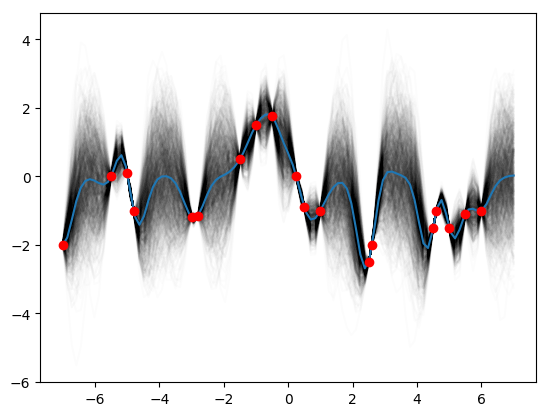Then finally (3, 1.16, 0.89):

K_star = k_star(3, 1.16, 0.89, x_star)
K_obs = k_obs(3, 1.16, 0.89,  x_obs)
K_obs_star = k_obs_star(3, 1.16, 0.89, x_obs, x_star)

post_mean = K_obs_star.T.dot(np.linalg.pinv(K_obs)).dot(y_obs)
post_var = K_star - K_obs_star.T.dot(np.linalg.pinv(K_obs)).dot(K_obs_star)

posteriors = st.multivariate_normal.rvs(mean=post_mean, cov=post_var, size=1000)

for i in range(1000):
plt.plot(x_star, posteriors[i], c='k', alpha=0.01)

plt.plot(x_star, posteriors.mean(axis=0))

plt.plot(x_obs, y_obs, 'ro')
plt.show()And there you have it! Figs 2.2, 2.4, and 2.5 from Rasmussen and Williams.